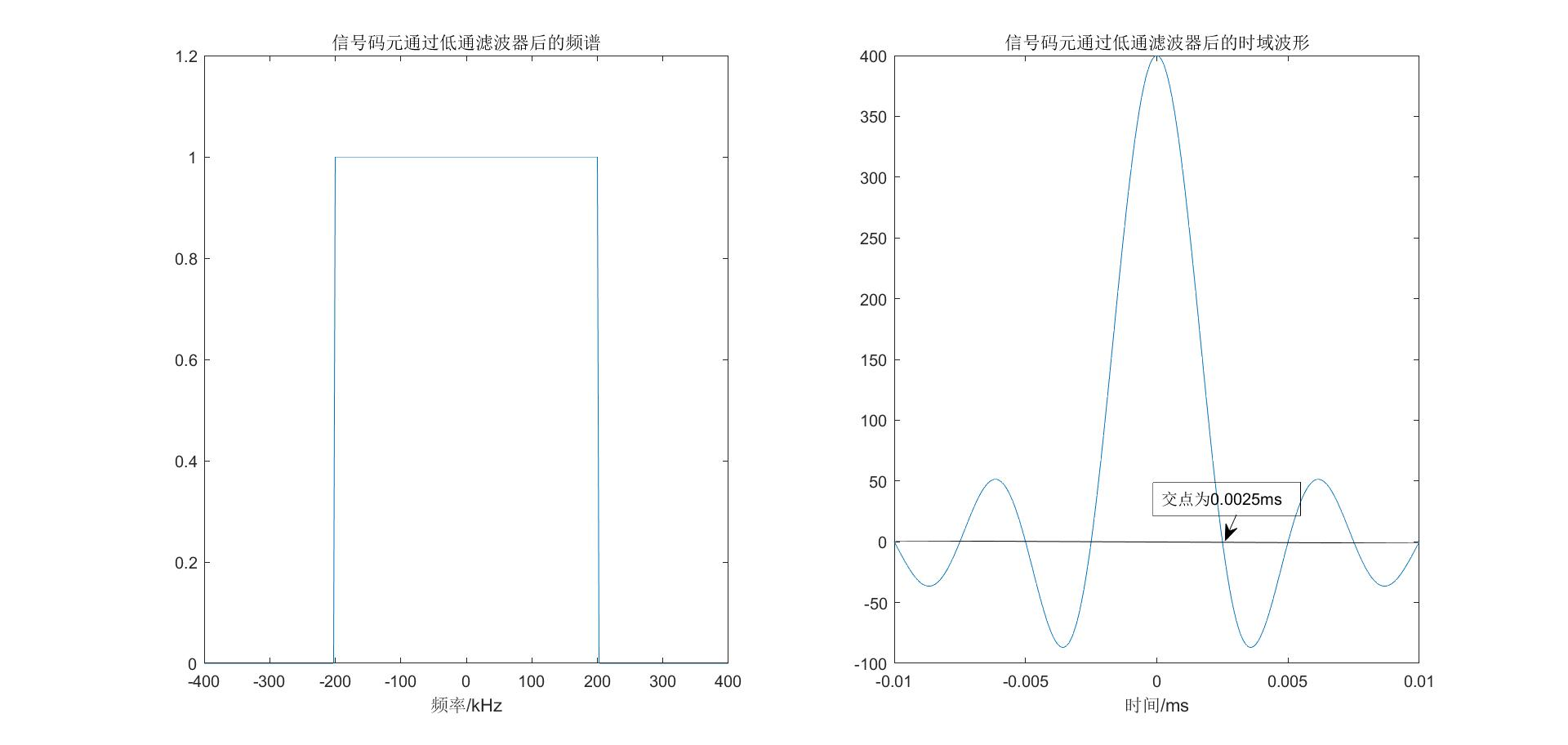# 奈奎斯特准则和香农公式的半定量解释

## 二、奈奎斯特准则

$$B_{max}=2W$$

$$C_{max}=B_{max}\times \log_2(M)=2W\log_2(M)$$
W理想低通的带宽，M为信号电平进制数，最简单的例子是码元只有0，1两个状态，一个码元包含1bit信息量。也可以是码元电平信号通过DA转换(数模转换器)成0，1/4，1/2，3/4四种电平，那么一个码元包含的信息量就是$\log_2 (4)=2 bit$。

### 奈奎斯特准则半定量解释5G通信之所以能够比4G快10倍，也是这个原因。4G的中心频段约2000MHz，频谱偏移1%的带宽资源为20M([1990,2010]MHz)，5G的商用中心频率为28GHz，频谱偏移1%的带宽资源为280M。也就是5G发送的信号码元的峰值更尖锐，码元间的时间间隔更短，每秒传输的码元更多，速率自然更快。

## 三、香农公式

$$C_{max}=W\log_2(1+S/N)$$
W是信道带宽，S是信源的平均功率，N是信道内高斯噪声功率。对比奈奎斯特公式，两者都有带宽，都有log，但是该怎么联系在一起呢？

### 香农公式的半定量解释

$$P=S+N \rightarrow V_T^2=V_S^2+V_N^2$$

$$M_{max}=V_T/V_N=\sqrt{V_T^2/V_N^2}=\sqrt{1+V_S^2/V_N^2}=\sqrt{ 1+S/N}$$

$$C_{max}=2W\log_2(M)=W\log_2(1+S/N)$$

## 参考资料

Shannon's Equation
《深入浅出理解通信原理》
《信号与系统》第二版 奥本海姆